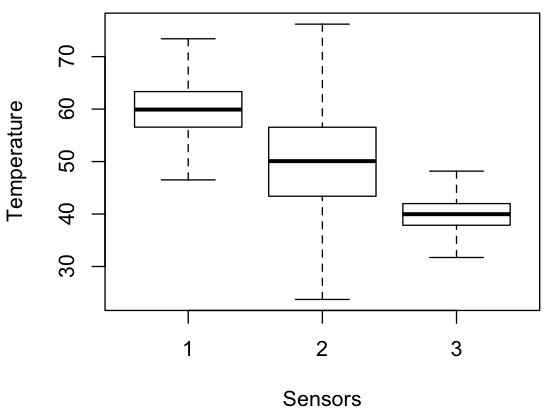# Introducing DataFu, an open source collection of useful Apache Pig UDFs

##### Matthew Hayes

At LinkedIn, we make extensive use of Apache Pig for performing data analysis on Hadoop. Pig is a simple, high-level programming language that consists of just a few dozen operators and makes it easy to write MapReduce jobs. For more advanced tasks, Pig also supports User Defined Functions (UDFs), which let you integrate custom code in Java, Python, and JavaScript into your Pig scripts.

Over time, as we worked on data intensive products such as People You May Know and Skills, we developed a large number of UDFs at LinkedIn. Today, I'm happy to announce that we have consolidated these UDFs into a single, general-purpose library called DataFu and we are open sourcing it under the Apache 2.0 license.

DataFu includes UDFs for common statistics tasks, PageRank, set operations, bag operations, and a comprehensive suite of tests. Read on to learn more.

### What's included?

Here's a taste of what you can do with DataFu:

### Example: Computing Quantiles

Let's walk through an example of how we could use DataFu. We will compute quantiles for a fake data set. You can grab all the code for this example, including scripts to generate test data, from this gist.

Let’s imagine that we collected 10,000 temperature readings from three sensors and have stored the data in HDFS under the name temperature.txt. The readings follow a normal distribution with mean values of 60, 50, and 40 degrees and standard deviation values of 5, 10, and 3.We can use DataFu to compute quantiles using the Quantile UDF. The constructor for the UDF takes the quantiles to be computed. In this case we provide 0.25, 0.5, and 0.75 to compute the 25th, 50th, and 75th percentiles (a.k.a quartiles). We also provide 0.0 and 1.0 to compute the min and max.

Quantile UDF example script:

``````define Quartile datafu.pig.stats.Quantile('0.0','0.25','0.5','0.75','1.0');

temperature = LOAD 'temperature.txt' AS (id:chararray, temp:double);

temperature = GROUP temperature BY id;

temperature_quartiles = FOREACH temperature {
sorted = ORDER temperature by temp; -- must be sorted
GENERATE group as id, Quartile(sorted.temp) as quartiles;
}

DUMP temperature_quartiles
``````

Quantile UDF example output, 10,000 measurements:

``````(1,(41.58171454288797,56.559375253601715,59.91093458980706,63.335574106080365,79.2841731889925))
(2,(14.393515179526304,43.39558395897533,50.081758806889766,56.54245916209963,91.03574746442487))
(3,(29.865710766927595,37.86257868882021,39.97075970657039,41.989584898364704,51.31349575866486))
``````

The values in each row of the output are the min, 25th percentile, 50th percentile (median), 75th percentile, and max.

### StreamingQuantile UDF

The Quantile UDF determines the quantiles by reading the input values for a key in sorted order and picking out the quantiles based on the size of the input DataBag. Alternatively we can estimate quantiles using the StreamingQuantile UDF, contributed to DataFu by Josh Wills of Cloudera, which does not require that the input data be sorted.

StreamingQuantile UDF example script:

``````define Quartile datafu.pig.stats.StreamingQuantile('0.0','0.25','0.5','0.75','1.0');

temperature = LOAD 'temperature.txt' AS (id:chararray, temp:double);

temperature = GROUP temperature BY id;

temperature_quartiles = FOREACH temperature {
-- sort not necessary
GENERATE group as id, Quartile(temperature.temp) as quartiles;
}

DUMP temperature_quartiles
``````

StreamingQuantile UDF example output, 10,000 measurements:

``````(1,(41.58171454288797,56.24183579452584,59.61727093346221,62.919576028265375,79.2841731889925))
(2,(14.393515179526304,42.55929349057328,49.50432161293486,56.020101184758644,91.03574746442487))
(3,(29.865710766927595,37.64744333815733,39.84941055349095,41.77693877565934,51.31349575866486))
``````

Notice that the 25th, 50th, and 75th percentile values computed by StreamingQuantile are fairly close to the exact values computed by Quantile.

### Accuracy vs. Runtime

StreamingQuantile samples the data with in-memory buffers. It implements the Accumulator interface, which makes it much more efficient than the Quantile UDF for very large input data. Where Quantile needs access to all the input data, StreamingQuantile can be fed the data incrementally. With Quantile, the input data will be spilled to disk as the DataBag is materialized if it is too large to fit in memory. For very large input data, this can be significant.

To demonstrate this, we can change our experiment so that instead of processing three sets of 10,000 measurements, we will process three sets of 1 billion. Let’s compare the output of Quantile and StreamingQuantile on this data set:

Quantile UDF example output, 1 billion measurements:

``````(1,(30.524038,56.62764,60.000134,63.372384,90.561695))
(2,(-9.845137,43.25512,49.999536,56.74441,109.714687))
(3,(21.564769,37.976644,40.000025,42.023622,58.057268))
``````

StreamingQuantile UDF example output, 1 billion measurements:

``````(1,(30.524038,55.993967,59.488968,62.775554,90.561695))
(2,(-9.845137,41.95725,48.977708,55.554239,109.714687))
(3,(21.564769,37.569332,39.692373,41.666762,58.057268))
``````

The 25th, 50th, and 75th percentile values computed using StreamingQuantile are only estimates, but they are pretty close to the exact values computed with Quantile. With StreamingQuantile and Quantile there is a tradeoff between accuracy and runtime. The script using Quantile takes 5 times as long to run as the one using StreamingQuantile when the input is the three sets of 1 billion measurements.

### Testing

DataFu has a suite of unit tests for each UDF. Instead of just testing the Java code for a UDF directly, which might overlook issues with the way the UDF works in an actual Pig script, we used PigUnit to do our testing. This let us run Pig scripts locally and still integrate our tests into a framework such as JUnit or TestNG.

We have also integrated the code coverage tracking tool Cobertura into our Ant build file. This helps us flag areas in DataFu which lack sufficient testing.

### Conclusion

We hope this gives you a taste of what you can do with DataFu. We are accepting contributions, so if you are interested in helping out, please fork the code and send us your pull requests!